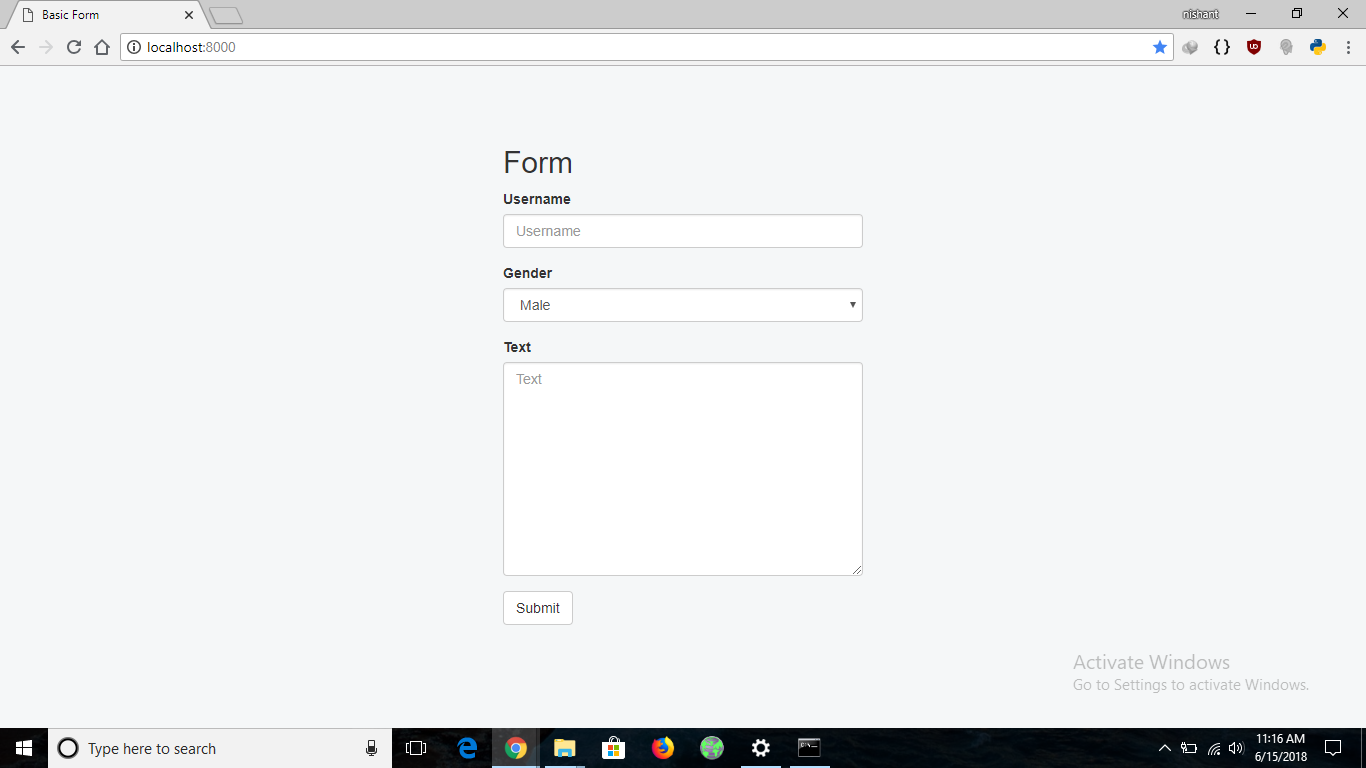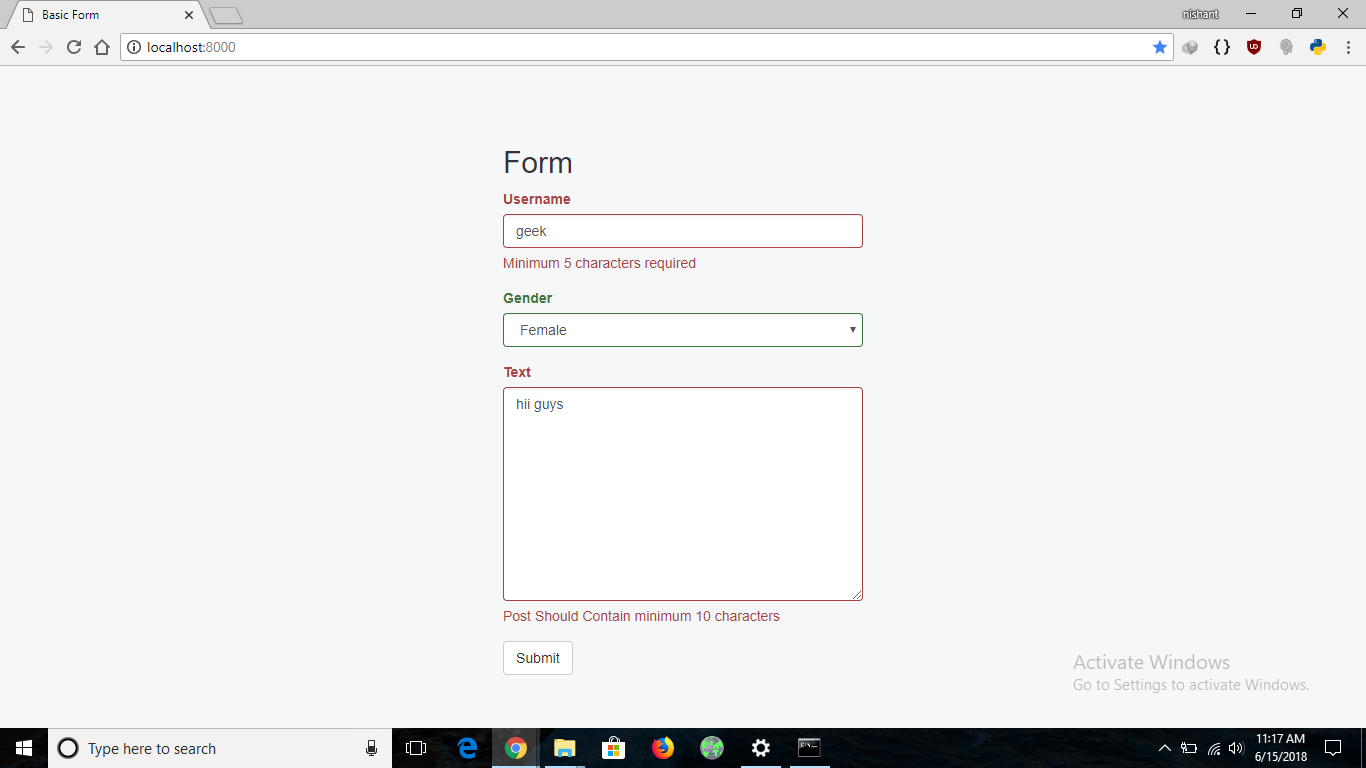# Python | Form validation using django

Prerequisites: Django Installation | Introduction to Django

Django works on a MVC pattern. So there is a need to create data models (or tables). For every table a model class is created. Some of the advantages of a MVC framework are listed out here.
Suppose there is a form which takes Username, gender and text as input from user, the task is to validate the data and save it.

In django this can be done, as follows:

 `from` `django.db ``import` `models ` ` `  `# model named Post ` `class` `Post(models.Model): ` `    ``Male ``=` `'M'` `    ``FeMale ``=` `'F'` `    ``GENDER_CHOICES ``=` `( ` `    ``(Male, ``'Male'``), ` `    ``(FeMale, ``'Female'``), ` `    ``) ` ` `  `    ``# define a username filed with bound  max length it can have ` `    ``username ``=` `models.CharField( max_length ``=` `20``, blank ``=` `False``, ` `                                 ``null ``=` `False``) ` `     `  `    ``# This is used to write a post ` `    ``text ``=` `models.TextField(blank ``=` `False``, null ``=` `False``) ` `     `  `    ``# Values for gender are restricted by giving choices ` `    ``gender ``=` `models.CharField(max_length ``=` `6``, choices ``=` `GENDER_CHOICES,  ` `                              ``default ``=` `Male) ` `     `  `    ``time ``=` `models.DateTimeField(auto_now_add ``=` `True``) `

After creating the data models, the changes need to be reflected in the database to do this run the following command:

`python manange.py makemigrations`

Doing this compiles the models and if it didn’t find any errors then, it creates a file in the migration folder. Later run the command given below to finally reflect the changes saved onto the migration file onto the database.

`python manage.py migrate`

Now a form can be created. Suppose that the username length should not be less than 5 and post length should be greater than 10. Then we define the Class PostForm with the required validation rules as follows:

 `from` `django.forms ``import` `ModelForm ` `from` `django ``import` `forms ` ` `  `# define the class of a form ` `from` `formValidationApp.models ``import` `*` `class` `PostForm(ModelForm): ` `    ``class` `Meta: ` `        ``# write the name of models for which the form is made ` `        ``model ``=` `Post         ` ` `  `        ``# Custom fields ` `        ``fields ``=``[``"username"``, ``"gender"``, ``"text"``] ` ` `  `    ``# this function will be used for the validation ` `    ``def` `clean(``self``): ` ` `  `        ``# data from the form is fetched using super function ` `        ``super``(PostForm, ``self``).clean() ` `         `  `        ``# extract the username and text field from the data ` `        ``username ``=` `self``.cleaned_data.get(``'username'``) ` `        ``text ``=` `self``.cleaned_data.get(``'text'``) ` ` `  `        ``# conditions to be met for the username length ` `        ``if` `len``(username) < ``5``: ` `            ``self``._errors[``'username'``] ``=` `self``.error_class([ ` `                ``'Minimum 5 characters required'``]) ` `        ``if` `len``(text) <``10``: ` `            ``self``._errors[``'text'``] ``=` `self``.error_class([ ` `                ``'Post Should Contain minimum 10 characters'``]) ` ` `  `        ``# return any errors if found ` `        ``return` `self``.cleaned_data `

Till now, the data models and the Form class are defined. Now the focus will be on how these modules, defined above, are actually used.

First run the localhost through this command

`python manage.py runserver`

Open http://localhost:8000/ in the browser, then it’s going to search in the urls.py file, looking for ‘ ‘ path

urls.py file is as given below:

 `from` `django.contrib ``import` `admin ` `from` `django.urls ``import` `path, include ` `from` `django.conf.urls ``import` `url ` `from` `django.shortcuts ``import` `HttpResponse ` `from` `. ``import` `views ` ` `  ` `  `urlpatterns ``=` `[ ` `    ``path('``', views.home, name ='``index'), ` `] `

Basically, this associates the ‘ ‘ url with a function home which is defined in views.py file.

views.py file:

 `from` `.models ``import` `Post ` `from` `.forms ``import` `PostForm ` `from` `.``import` `views ` `from` `django.shortcuts ``import` `HttpResponse, render, redirect ` ` `  ` `  `def` `home(request): ` ` `  `    ``# check if the request is post  ` `    ``if` `request.method ``=``=``'POST'``:   ` ` `  `        ``# Pass the form data to the form class ` `        ``details ``=` `PostForm(request.POST) ` ` `  `        ``# In the 'form' class the clean function  ` `        ``# is defined, if all the data is correct  ` `        ``# as per the clean function, it returns true ` `        ``if` `details.is_valid():   ` ` `  `            ``# Temporarily make an object to be add some ` `            ``# logic into the data if there is such a need ` `            ``# before writing to the database    ` `            ``post ``=` `details.save(commit ``=` `False``) ` ` `  `            ``# Finally write the changes into database ` `            ``post.save()   ` ` `  `            ``# redirect it to some another page indicating data ` `            ``# was inserted successfully ` `            ``return` `HttpResponse(``"data submitted successfully"``) ` `             `  `        ``else``: ` `         `  `            ``# Redirect back to the same page if the data ` `            ``# was invalid ` `            ``return` `render(request, ``"home.html"``, {``'form'``:details})   ` `    ``else``: ` ` `  `        ``# If the request is a GET request then, ` `        ``# create an empty form object and  ` `        ``# render it into the page ` `        ``form ``=` `PostForm(``None``)    ` `        ``return` `render(request, ``'home.html'``, {``'form'``:form}) `

home.html template file

 `{% load bootstrap3 %} ` `{% bootstrap_messages %} ` ` ` `<``html` `lang``=``"en"``> ` ` `  `<``head` `> ` ` `  `    ``<``title``>Basic Form ` `     `  `    ``<``meta` `charset``=``"utf-8"` `/> ` `     `  `    ``<``meta` `name``=``"viewport"` `content``=``"width=device-width, initial-scale=1, shrink-to-fit=no"``> ` ` `  `<``link` `rel``=``"stylesheet"` `href``=``"https://maxcdn.bootstrapcdn.com/bootstrap/3.3.7/css/bootstrap.min.css"``> ` `<``script` `src``=``"https://ajax.googleapis.com/ajax/libs/jquery/3.2.1/jquery.min.js"``> ` ` ` `<``script` `src``=``"https://maxcdn.bootstrapcdn.com/bootstrap/3.3.7/js/bootstrap.min.js"``> ` ` ` ` ` ` `  `<``body`  `style``=``"padding-top: 60px;background-color: #f5f7f8 !important;"``> ` `    ``<``div` `class``=``"container"``> ` `    ``<``div` `class``=``"row"``> ` `        ``<``div` `class``=``"col-md-4 col-md-offset-4"``> ` `        ``<``h2``>Form ` `             ``<``form` `action``=``""` `method``=``"post"``><``input` `type``=``'hidden'``/> ` `             ``{%csrf_token %} ` ` `  `                ``{% bootstrap_form form %} ` ` ` `              ``<``div` `class``=``"form-group"``> ` `                ``<``button` `type``=``"submit"` `class``=``"btn btn-default "``> ` `                  ``Submit ` `                `` ` ` `  `                  `` ` ` `  `                `` ` `        `` ` `    `` ` ` ` ` `  ` ` ` `  ` `

Opening http://localhost:8000/ in the browser shows the following,If a form with username of length less than 5 is submitted, it gives an error at the time of submission and similarly for the Post Text filled as well. The following image shows how the form behaves on submitting in valid form data.My Personal Notes arrow_drop_upCheck out this Author's contributed articles.

If you like GeeksforGeeks and would like to contribute, you can also write an article using contribute.geeksforgeeks.org or mail your article to contribute@geeksforgeeks.org. See your article appearing on the GeeksforGeeks main page and help other Geeks.

Please Improve this article if you find anything incorrect by clicking on the "Improve Article" button below.

Improved By : Akanksha_Rai

Article Tags :

2

Please write to us at contribute@geeksforgeeks.org to report any issue with the above content.